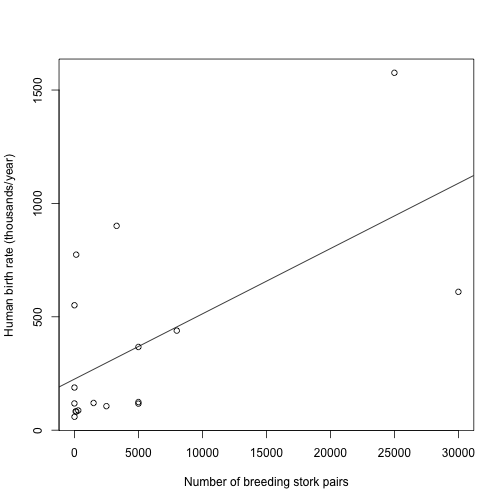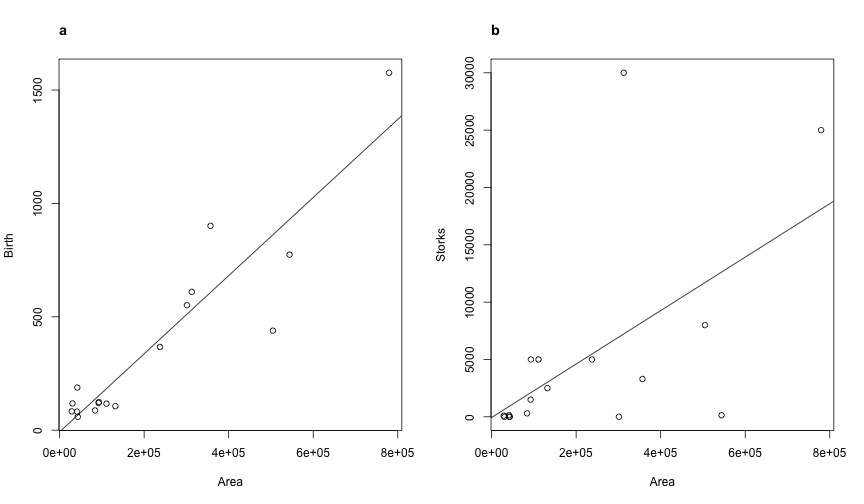### Sources

#### Data

Storks (`"storks.csv"`), a comma separated file with number of storks, human birth rate, and area size in 17 European countries (From Matthews 2000)

### Codes

#### The baby-delivering storks example

After downloading the `storks.csv` file to your R working directory, you can explore this data by yourself uploading it to R as a dataframe (which we will call `storks.dat`):

``````storks.dat <- read.csv("storks.csv")
str(storks.dat)
``````
``````'data.frame':   17 obs. of  5 variables:
\$ Country: Factor w/ 17 levels "Albania","Austria",..: 1 2 3 4 5 6 7 8 9 10 ...
\$ Area   : int  28750 83860 30520 111000 43100 544000 357000 132000 41900 93000 ...
\$ Storks : int  100 300 1 5000 9 140 3300 2500 4 5000 ...
\$ Humans : num  3.2 7.6 9.9 9 5.1 56 78 10 15 11 ...
\$ Birth  : int  83 87 118 117 59 774 901 106 188 124 ...
``````

You can now reproduce figure 2 of the chapter with the following script:

``````with(storks.dat, plot(Birth ~ Storks, xlab = "Number of breeding stork pairs",
ylab = "Human birth rate (thousands/year)"))
storks.lm <- lm(Birth ~ Storks, data = storks.dat)
abline(storks.lm)
``````Verify by yourself the relationship between number of storks (Storks) and human birth rates (Birth) looking at the summary of the linear model:

``````summary(storks.lm)
``````
``````
Call:
lm(formula = Birth ~ Storks, data = storks.dat)

Residuals:
Min     1Q Median     3Q    Max
-479   -166   -145     -2    631

Coefficients:
Estimate Std. Error t value Pr(>|t|)
(Intercept) 225.0287    93.5606    2.41   0.0295 *
Storks        0.0288     0.0094    3.06   0.0079 **
---
Signif. codes:  0 '***' 0.001 '**' 0.01 '*' 0.05 '.' 0.1 ' ' 1

Residual standard error: 332 on 15 degrees of freedom
Multiple R-squared:  0.385, Adjusted R-squared:  0.344
F-statistic: 9.38 on 1 and 15 DF,  p-value: 0.0079
``````

Let's now reproduce figure 3a-b to explore the bivariate relationships between Birth rate and country area (Area; Fig. 3a) and between number of storks and Area (Fig. 3b):

``````par(mfrow = c(1, 2))

with(storks.dat, plot(Birth ~ Area))
storks2.lm <- lm(Birth ~ Area, data = storks.dat)
abline(storks2.lm)

with(storks.dat, plot(Storks ~ Area))
storks3.lm <- lm(Storks ~ Area, data = storks.dat)
abline(storks3.lm)
``````Country area seems indeed to be strongly correlated both to human birth rate and the number of stork pairs. Let's thus see what happens when we statistically control for the effect of the confounding variable Area when we include this variable in the model of the relationship between Storks and Birth:

``````summary(lm(Birth ~ Area + Storks, data = storks.dat))
``````
``````
Call:
lm(formula = Birth ~ Area + Storks, data = storks.dat)

Residuals:
Min     1Q Median     3Q    Max
-400.7  -57.5  -27.9   77.1  323.4

Coefficients:
Estimate Std. Error t value Pr(>|t|)
(Intercept) -7.411687  56.702180   -0.13     0.90
Area         0.001583   0.000227    6.96  6.6e-06 ***
Storks       0.005995   0.005651    1.06     0.31
---
Signif. codes:  0 '***' 0.001 '**' 0.01 '*' 0.05 '.' 0.1 ' ' 1

Residual standard error: 163 on 14 degrees of freedom
Multiple R-squared:  0.862, Adjusted R-squared:  0.842
F-statistic: 43.8 on 2 and 14 DF,  p-value: 9.45e-07
``````

In the above output check how the significance of the effect of the number of stork pairs changes when we statistically control for Area. Go back to Section 8.2 of Chapter 8 for a discussion of these results.

#### Testing causality in the baby-delivering storks example

In fig.8.4 of the book, we represented graphically a possible causal model of the relationship between the number of breeding pairs of storks (Storks), human birthrates in European countries (Birth), country area (Area) and human population size (Human). The d-separation statements which define this causal model are the following (see section 8.3 for details):

(Storks, Birth){Area}
(Storks, Humans){Area, Birth}
(Area, Humans){Birth}

to test these d-separation statements, we translate them in the following three linear models:

Birth ~ Area + Storks
Humans ~ Area + Birth + Storks
Humans ~ Birth + Area

Which can be run in R as follows:

``````m1 <- lm(Birth ~ Area + Storks, data = storks.dat)
m2 <- lm(Humans ~ Area + Birth + Storks, data = storks.dat)
m3 <- lm(Humans ~ Birth + Area, data = storks.dat)
``````

We now can save the p-values of the 3 partial regressions we are testing:

``````p1.m1 <- summary(m1)\$coefficients["Storks", 4]
p2.m2 <- summary(m2)\$coefficients["Storks", 4]
p3.m3 <- summary(m3)\$coefficients["Area", 4]
``````

What are the p-values of these 3 partial regression coefficients? To get an idea of the goodness of fit of the proposed path model to the data we can calculate Fisher's C statistic using the following formula (see formula 8.1 in Chapter 8):

``````C <- -2 * (log(p1.m1) + log(p2.m2) + log(p3.m3))
C
``````
`````` 7.715
``````

Following the text you can see that if the C-statistic is significant (i.e. the p-value is < 0.05) we can reject the hypothesis that the model presents a good fit to the data or in other words that the observed correlation structure could be caused by the proposed causal model.

To calculate the p-value of the C statistics we must first determine the number of degrees of freedom. This is simply 2 times the number of conditional independencies (in this case = 3). We can thus calculate the p-value of the C statistic as follows:

``````C.pval <- 1 - pchisq(C, 2 * 3)
C.pval
``````
`````` 0.2597
``````

If you still believe that storks deliver babies, you can try an alternative model in which instead of having a direct causal link from Area to Birth rate, you have a direct causal link from the number of Stork pairs to Birth rate, while the other relationships stay the same as in the previous model. This alternative causal model is depicted as a DAG in Figure 8.5.

Following formula 8.2 in Chapter 8, we can calculate the numer of conditional independencies in the minimal set counting the number of vertices (V) and the number of arrows (A) in the directed acyclic graph. In the DAG represented in fig. 8.5 we have 4 vertices and 3 arrows, and thus:

``````V <- 4
A <- 3
(factorial(V)/(2 * factorial(V - 2))) - A
``````
`````` 3
``````

We will use this formula again later, so it is easier just to define a new function to carry out this task for us in future (which we will call `condNum`):

``````condNum <- function(V, A) {
(factorial(V)/(2 * factorial(V - 2))) - A
}
``````

A simple call to `condNum`, providing the number of Vertices and the number of Arrows (in this order!) as arguments, will give us the right answer:

``````condNum(4, 3)
``````
`````` 3
``````

We see the minimal set contains 3 conditional independencies, which are:

(Area,Birth) {Storks}
(Area,Humans) {Birth}
(Storks, Humans) {Birth}

These translate into the following linear models:

``````m2.1 <- lm(Birth ~ Storks + Area, data = storks.dat)
m2.2 <- lm(Humans ~ Birth + Area, data = storks.dat)
m2.3 <- lm(Humans ~ Birth + Storks, data = storks.dat)
``````

The p-values which we will need to calculate the C statistics for this causal model will be:

``````p1.m2.1 <- summary(m2.1)\$coefficients["Area", 4]
p2.m2.2 <- summary(m2.2)\$coefficients["Area", 4]
p3.m2.3 <- summary(m2.3)\$coefficients["Storks", 4]
``````

Following the instructions above you can now calculate by yourself the C statistics and its associated p-value for this alternative causal model. Do you still believe that storks deliver babies?

### References

• Matthews, R. 2000. Storks deliver babies (p=0.008). Teaching Statistics 22:36-38.